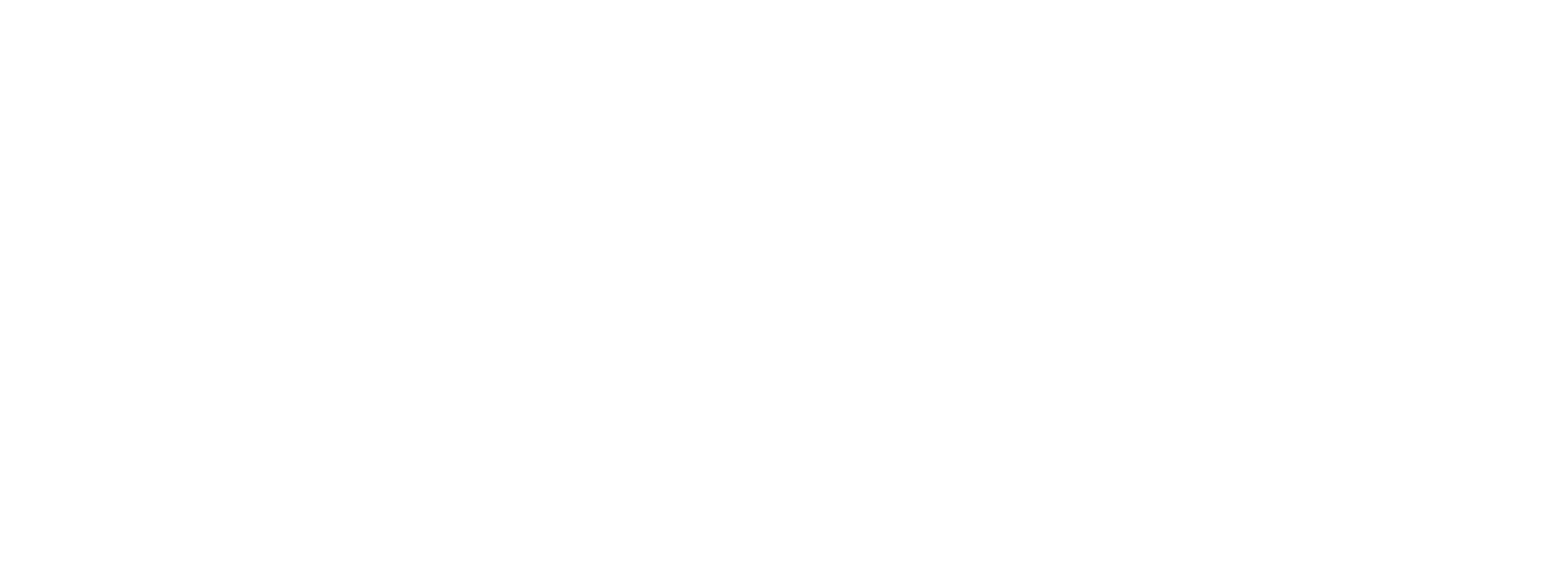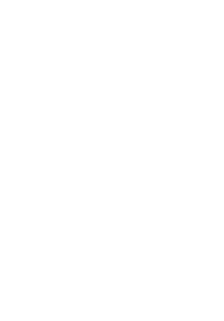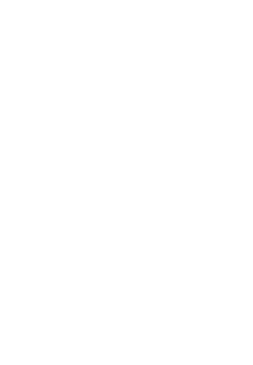# Human Pose Estimation using OpenCV

3
3863## Introduction To Human Pose Estimation using OpenCV

In This article, we will discuss how to use a Deep learning network model for Human Pose Estimation using OpenCV. For this task we used the pre-trained model name Caffe model that won the COCO keypoints challenge in 2016 in our own application.

NOTE : You will need OpenCV version 4.1.2 and above to run the code.

## Human Pose Estimation using OpenCV

In computer vision where we detect the position and orientation of an object. This means detecting keypoints location of particular objects.

For example, in the problem of face pose estimation(i.e. Facial landmark detection ), this means we detect a human face.

A related problem is Head pose estimation where we use the facial landmarks to obtain the 3D orientation of a human head with respect to the camera.

In this article, we will work on Human Pose Estimation using OpenCV, where to detect and localize the major parts of the body like joint, shoulder, knee, wrist, etc.

This article, we will solve a simpler problem of detecting keypoints on the body.

Remember the movie scene  where Tobey Maguire wears the Spider Man suit using gestures?

Now such a type of suit is ever built, it would require human pose estimation!

Figure1:- Sample figure of Pose Estimation

## Key Point Pose Detection

This field was little progress in pose estimation because of the lack of datasets. AI requires good datasets with proper quality. Some challenging datasets have been released in the last few years which have made it easier for researchers in the last few years.

Some of the datasets are :

1. Multi-Person Pose Estimation Model

Let’s briefly go over the architecture before we explain how to use the pre-trained model.

Widget not in any sidebars

## Architecture Overview

The model takes as input a color image of size w × h and produces, as output, the 2D locations of keypoints for each person in the image. The detection takes place in three stages :

1. Stage 0: The first 10 layers of the VGGNet are used to create feature maps for the input image.
2. Stage 1: A 2-branch multistage CNN is used where the first branch predicts a set of 2D confidence maps (S) of body part locations ( e.g. elbow, knee etc.). Given below are confidence maps and Affinity maps for the keypoint – Left Shoulder.
3. Stage 2: The confidence and affinity maps are parsed by greedy inference to produce the 2D key points for all people in the image.

This architecture won the COCO keypoints challenge

3.2 Pre-trained models for Human Pose Estimation

The authors of the paper have shared two models – one is trained on the Multi-Person Dataset ( MPII ) and the other is trained on the COCO dataset. The COCO model produces 18 points, while the MPII model outputs 15 points. The output plotted on a person is shown in the image below.

COCO Output Format Nose – 0, Neck – 1, Right Shoulder – 2, Right Elbow – 3, Right Wrist – 4, Left Shoulder – 5, Left Elbow – 6, Left Wrist – 7, Right Hip – 8, Right Knee – 9, Right Ankle – 10, Left Hip – 11, Left Knee – 12, LAnkle – 13, Right Eye – 14, Left Eye – 15, Right Ear – 16, Left Ear – 17, Background – 18

MPII Output Format Head – 0, Neck – 1, Right Shoulder – 2, Right Elbow – 3, Right Wrist – 4, Left Shoulder – 5, Left Elbow – 6, Left Wrist – 7, Right Hip – 8, Right Knee – 9, Right Ankle – 10, Left Hip – 11, Left Knee – 12, Left Ankle – 13, Chest – 14, Background – 15

``
1. Tutorial

In this section, we will see how to load the trained models in OpenCV and check the outputs. We will discuss code for only single person pose estimation to keep things simple. These outputs can be used to find the pose for every person in a frame if multiple people are present.

`Widget not in any sidebars`

There are separate files for Image and Video inputs.

Future Work: We will cover the multiple-person case in a future post.

Use the getModels.sh file provided with the code to download all the model weights to the respective folders. Note that the configuration proto files are already present in the folders.

sudo chmod a+x getModels.sh

./getModels.sh

We are using models trained on Caffe Deep Learning Framework. Caffe models have 2 files –

1. prototxt file which specifies the architecture of the neural network – how the different layers are arranged etc.
2. .caffemodel file which stores the weights of the trained model

We will use these two files to load the network into memory.

``````# Specify the paths for the 2 files
protoFile = "pose/mpi/pose_deploy_linevec_faster_4_stages.prototxt"
weightsFile = "pose/mpi/pose_iter_160000.caffemodel"
# Read the network into Memory
``````

Step 3: Read the image and Prepare Input to the network

The input frame that we read using OpenCV should be converted to an input blob ( like Caffe ) so that it can be fed to the network. This is done using the blobFromImage function which converts the image from OpenCV format to Caffe blob format. The parameters are to be provided in the blobFromImage function. First we normalize the pixel values to be in (0,1). Then we specify the dimensions of the image. Next, the Mean value to be subtracted, which is (0,0,0). There is no need to swap the R and B channels since both OpenCV and Caffe use BGR format.

``````# Read image
# Specify the input image dimensions
inWidth = 368
inHeight = 368
# Prepare the frame to be fed to the network
inpBlob = cv2.dnn.blobFromImage(frame, 1.0 / 255, (inWidth, inHeight), (0, 0, 0), swapRB=False, crop=False)

# Set the prepared object as the input blob of the network
net.setInput(inpBlob)
``````

Step 4: Make Predictions and Parse Key Points

Once the image is passed to the model, the predictions can be made using a single line of code. The forward method for the DNN class in OpenCV makes a forward pass through the network which is just another way of saying it is making a prediction.

The output is a 4D matrix :

1. The first dimension being the image ID ( in case you pass more than one image to the network ).
2. The second dimension indicates the index of a keypoint. The model produces Confidence Maps and Part Affinity maps which are all concatenated. For COCO model it consists of 57 parts – 18 keypoint confidence Maps + 1 background + 19*2 Part Affinity Maps. Similarly, for MPI, it produces 44 points. We will be using only the first few points which correspond to Keypoints.
3. The third dimension is the height of the output map.
4. The fourth dimension is the width of the output map.

We check whether each keypoint is present in the image or not. We get the location of the keypoint by finding the maxima of the confidence map of that keypoint. We also use a threshold to reduce false detections.

Once the keypoints are detected, we just plot them on the image.

``````H = out.shape
W = out.shape

# Empty list to store the detected keypoints

points = []

for i in range(len()):
# confidence map of corresponding body's part.
probMap = output[0, i, :, :]

# Find global maxima of the probMap.

minVal, prob, minLoc, point = cv2.minMaxLoc(probMap)

# Scale the point to fit on the original image
x = (frameWidth * point) / W
y = (frameHeight * point) / H

if prob &amp;gt; threshold :
cv2.circle(frame, (int(x), int(y)), 15, (0, 255, 255), thickness=-1, lineType=cv.FILLED)

cv2.putText(frame, "{}".format(i), (int(x), int(y)), cv2.FONT_HERSHEY_SIMPLEX, 1.4, (0, 0, 255), 3, lineType=cv2.LINE_AA)

# Add the point to the list if the probability is greater than the threshold

points.append((int(x), int(y)))

else :

points.append(None)

cv2.imshow("Output-Keypoints",frame)

cv2.waitKey(0)

cv2.destroyAllWindows()
``````Widget not in any sidebars

Step 5: Draw Skeleton

Since we know the indices of the points before-hand, we can draw the skeleton when we have the key points by just joining the pairs. This is done using the code given below.

``````for pair in POSE_PAIRS:
partA = pair
partB = pair
if points[partA] and points[partB]:
cv2.line(frameCopy, points[partA], points[partB], (0, 255, 0), 3)``````## Conclusion

It concludes that In computer vision where we detect the position and orientation of an object. We used a single image to detect the position.

1.rama
2.rama
3.rama